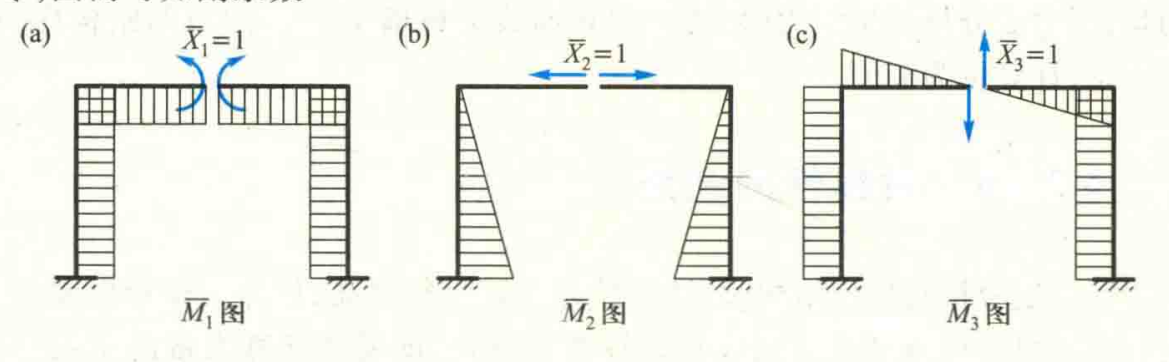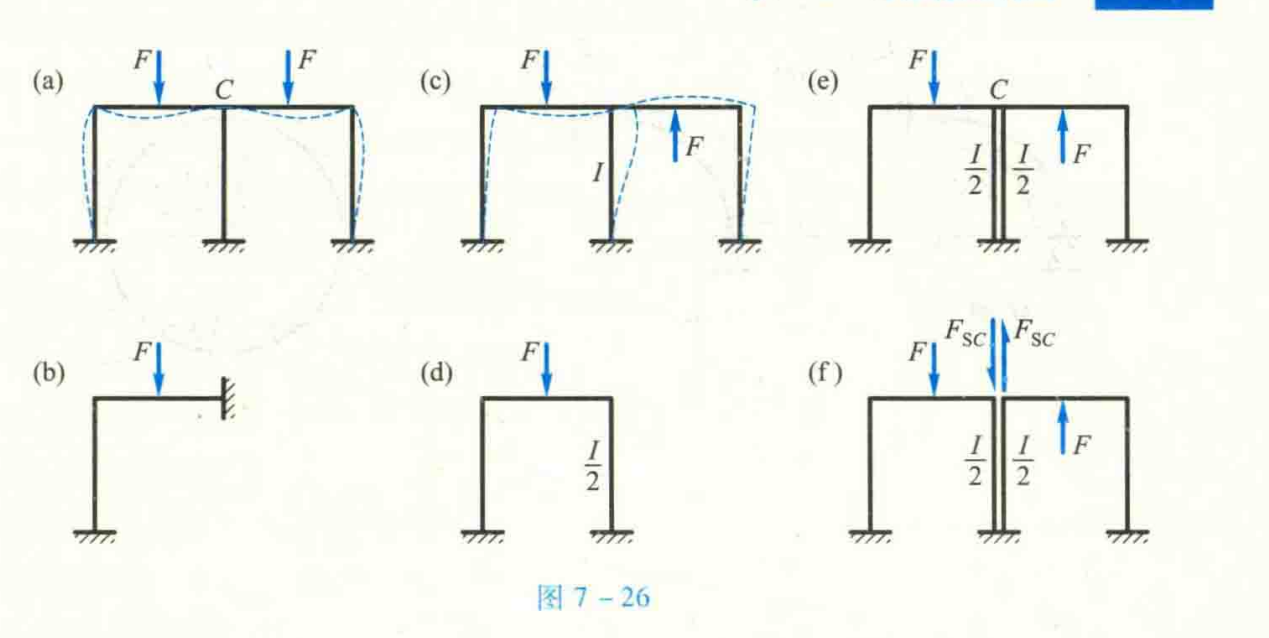# 结构力学-力法

## 概述

• $[X]$为假设的支座反力列向量，其等于${[{X_1},{X_2},...,{X_n}]^T}$；
• $[\delta ]$为各假设的支座反力当其大小等于单位力时，在对应的支座反力方向上引起的位移。其等于:
$$\left[ {\begin{array}{*{20}{c}} {\int {\frac{{{{\overline M }_{X1}}{{\overline M }_{X1}}}}{{EI}}} }&{\int {\frac{{{{\overline M }_{X1}}{{\overline M }_{X2}}}}{{EI}}} }&{...}&{\int {\frac{{{{\overline M }_{X1}}{{\overline M }_{Xn}}}}{{EI}}} }\\ {\int {\frac{{{{\overline M }_{X2}}{{\overline M }_{X1}}}}{{EI}}} }&{\int {\frac{{{{\overline M }_{X2}}{{\overline M }_{X2}}}}{{EI}}} }&{...}&{\int {\frac{{{{\overline M }_{X2}}{{\overline M }_{Xn}}}}{{EI}}} }\\ \vdots & \vdots & \ddots & \vdots \\ {\int {\frac{{{{\overline M }_{Xn}}{{\overline M }_{X1}}}}{{EI}}} }&{\int {\frac{{{{\overline M }_{Xn}}{{\overline M }_{X2}}}}{{EI}}} }&{...}&{\int {\frac{{{{\overline M }_{Xn}}{{\overline M }_{Xn}}}}{{EI}}} } \end{array}} \right]$$

• $[\Delta P]$为外荷载所引起的对应位置的位移列向量，其等于：${\left[ {\int {\frac{{{M_p}{{\overline M }_{X1}}}}{{EI}},} \int {\frac{{{M_p}{{\overline M }_{X2}}}}{{EI}},...,\int {\frac{{{M_p}{{\overline M }_{Xn}}}}{{EI}}} } } \right]^T}$
• 其中$M_p$为所有的外荷载对于结构内力的组合效应。

## 原理

### 力法的典型方程

$$\begin{array}{l} {S_{X1}} + \Delta P = 0\\ 其中{S_{X1}} = {X_1}{\delta _{11}};\\ \Delta P = P{\delta _{1P}} \end{array}$$
• ${\delta _{11}}$为假定的单位支座反力在该方向上引起的位移；
• ${\delta _{1P}}$为外荷载综合作用在该支座反力方向上引起的位移### 多支座反力的线性方程和求解

$$\left[ {\begin{array}{*{20}{c}} {{\delta _{11}}}&{{\delta _{12}}}&{...}&{{\delta _{1n}}}\\ {{\delta _{21}}}&{{\delta _{22}}}&{...}&{{\delta _{2n}}}\\ \vdots & \vdots & \ddots & \vdots \\ {{\delta _{n1}}}&{{\delta _{n2}}}&{...}&{{\delta _{nn}}} \end{array}} \right]$$

$${\begin{array}{*{20}{c}} {{\delta _{11}} = \int {\frac{{{{\overline M }_{X1}}{{\overline M }_{X1}}}}{{EI}}} }&{{\delta _{12}} = \int {\frac{{{{\overline M }_{X1}}{{\overline M }_{X2}}}}{{EI}}} }&{...}&{{\delta _{1n}} = \int {\frac{{{{\overline M }_{X1}}{{\overline M }_{Xn}}}}{{EI}}} }\\ {{\delta _{21}} = \int {\frac{{{{\overline M }_{X2}}{{\overline M }_{X1}}}}{{EI}}} }&{{\delta _{22}} = \int {\frac{{{{\overline M }_{X2}}{{\overline M }_{X2}}}}{{EI}}} }&{...}&{{\delta _{2n}} = \int {\frac{{{{\overline M }_{X2}}{{\overline M }_{Xn}}}}{{EI}}} }\\ \vdots & \vdots & \ddots & \vdots \\ {{\delta _{n1}} = \int {\frac{{{{\overline M }_{Xn}}{{\overline M }_{X1}}}}{{EI}}} }&{{\delta _{n2}} = \int {\frac{{{{\overline M }_{Xn}}{{\overline M }_{X2}}}}{{EI}}} }&{...}&{{\delta _{nn}} = \int {\frac{{{{\overline M }_{Xn}}{{\overline M }_{Xn}}}}{{EI}}} } \end{array}}$$

$${\left[ {\Delta {P_1} = \int {\frac{{{M_p}{{\bar M}_{X1}}}}{{EI}},} \Delta {P_2} = \int {\frac{{{M_p}{{\bar M}_{X2}}}}{{EI}},...,\Delta {P_n}\int {\frac{{{M_p}{{\bar M}_{Xn}}}}{{EI}}} } } \right]^T}$$

</div>

$$\left\{ {\begin{array}{*{20}{c}} {{X_1}{\delta _{11}} + {X_2}{\delta _{21}} + ... + {X_n}{\delta _{n1}} + \Delta {P_1} = 0}\\ {{X_2}{\delta _{12}} + {X_2}{\delta _{22}} + ... + {X_n}{\delta _{n2}} + \Delta {P_2} = 0}\\ \vdots \\ {{X_n}{\delta _{1n}} + {X_2}{\delta _{2n}} + ... + {X_n}{\delta _{nn}} + \Delta {P_n} = 0} \end{array}} \right.$$

## 基于力法的对称性求解的应用

1. 结构几何对称
2. 结构的刚度也互相对称

### 例子

#### 正对称$$\begin{array}{l} {\delta _{13}} = {\delta _{31}} = 0,{\delta _{23}} = {\delta _{32}} = 0\\ \left\{ {\begin{array}{*{20}{c}} {{\delta _{11}}{X_1} + {\delta _{12}}{X_2} + {\Delta _{1P}} = 0}\\ {{\delta _{21}}{X_1} + {\delta _{22}}{X_2} + {\Delta _{2P}} = 0}\\ {{\delta _{33}}{X_3} + {\Delta _{3P}} = 0} \end{array}} \right. \end{array}$$

#### 反对称

1. 奇数跨对称刚架：1. 偶数跨对称刚架## 参考资料

https://www.eatrice.cn/post/结构力学-力法/

2020年5月10日